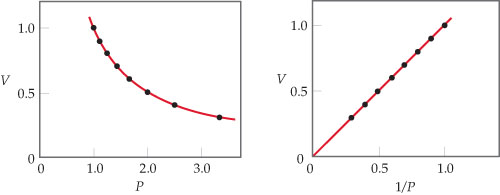# Problem: Boyles Law: For a fixed quantity of gas at constant temperature, the volume of the gas is inversely proportional to its pressure.What would a plot of P versus 1/V look like for a fixed quantity of gas at a fixed temperature?

###### FREE Expert Solution

Recall that the ideal gas law is:

$\overline{){\mathbf{PV}}{\mathbf{=}}{\mathbf{nRT}}}$

The pressure and volume of a gas are related to the number of moles, gas constant and temperatureThe value nRT is constant

$\overline{){\mathbf{PV}}{\mathbf{=}}{\mathbf{constant}}}$###### Problem DetailsBoyles Law: For a fixed quantity of gas at constant temperature, the volume of the gas is inversely proportional to its pressure.

What would a plot of P versus 1/V look like for a fixed quantity of gas at a fixed temperature?